# Coulombs Law Chemical Bonding IV A Coulombs Law

• Slides: 13Coulomb’s Law Chemical Bonding IV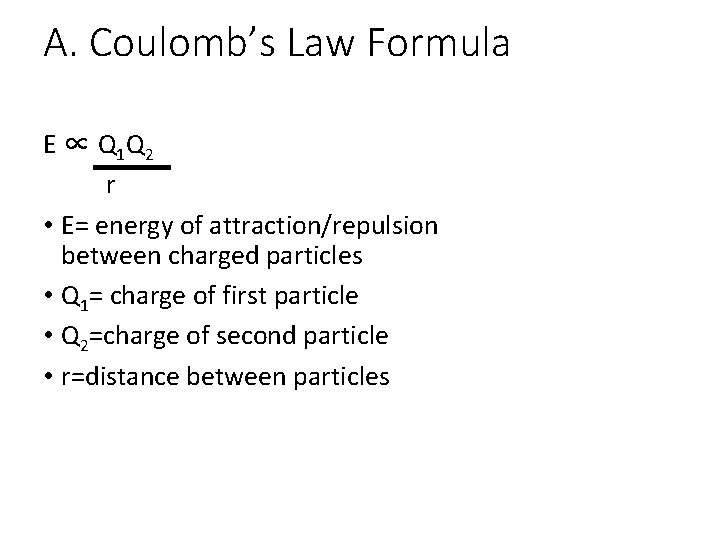A. Coulomb’s Law Formula E ∝ Q 1 Q 2 r • E= energy of attraction/repulsion between charged particles • Q 1= charge of first particle • Q 2=charge of second particle • r=distance between particles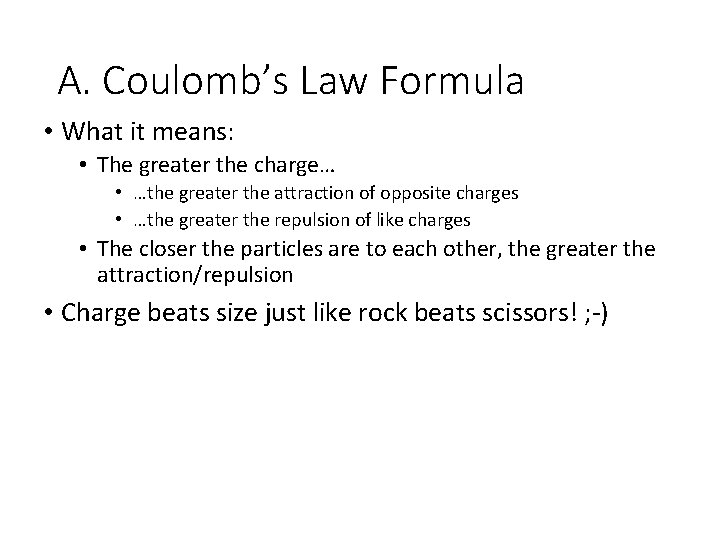A. Coulomb’s Law Formula • What it means: • The greater the charge… • …the greater the attraction of opposite charges • …the greater the repulsion of like charges • The closer the particles are to each other, the greater the attraction/repulsion • Charge beats size just like rock beats scissors! ; -)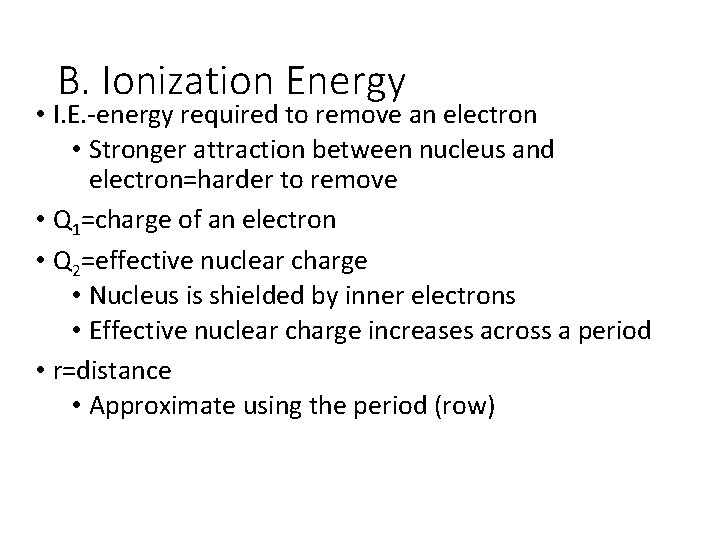B. Ionization Energy • I. E. -energy required to remove an electron • Stronger attraction between nucleus and electron=harder to remove • Q 1=charge of an electron • Q 2=effective nuclear charge • Nucleus is shielded by inner electrons • Effective nuclear charge increases across a period • r=distance • Approximate using the period (row)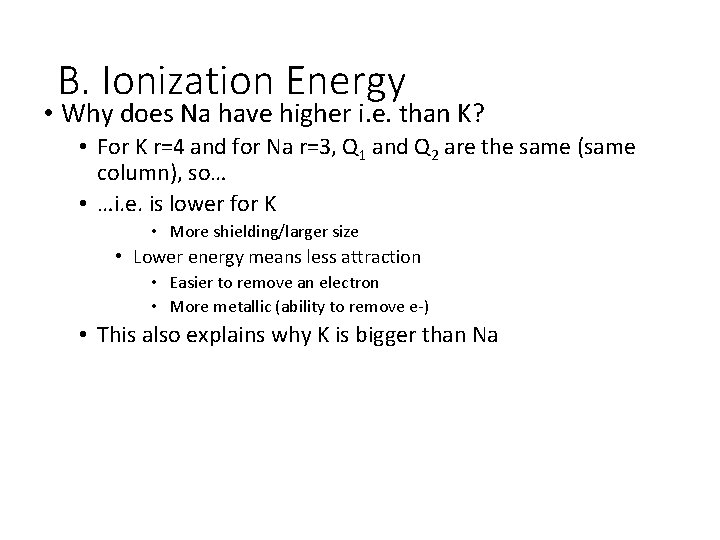B. Ionization Energy • Why does Na have higher i. e. than K? • For K r=4 and for Na r=3, Q 1 and Q 2 are the same (same column), so… • …i. e. is lower for K • More shielding/larger size • Lower energy means less attraction • Easier to remove an electron • More metallic (ability to remove e-) • This also explains why K is bigger than Na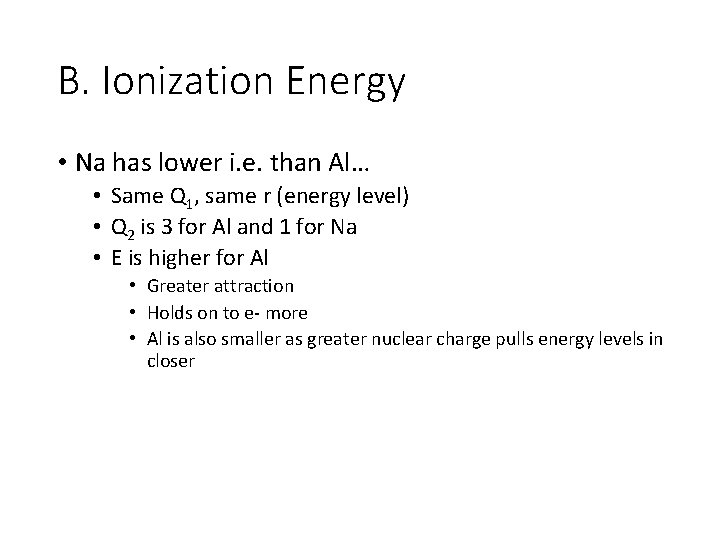B. Ionization Energy • Na has lower i. e. than Al… • Same Q 1, same r (energy level) • Q 2 is 3 for Al and 1 for Na • E is higher for Al • Greater attraction • Holds on to e- more • Al is also smaller as greater nuclear charge pulls energy levels in closerC. Lattice Energy and Bonding • E=Lattice Energy: energy required to separate oppositely charged ions • Higher lattice energy=stronger bond • Q 1=charge of first ion • Q 2=charge of second ion • r=bond length: distance between nuclei • Can approximate using period of element that is isoelectronic with that ion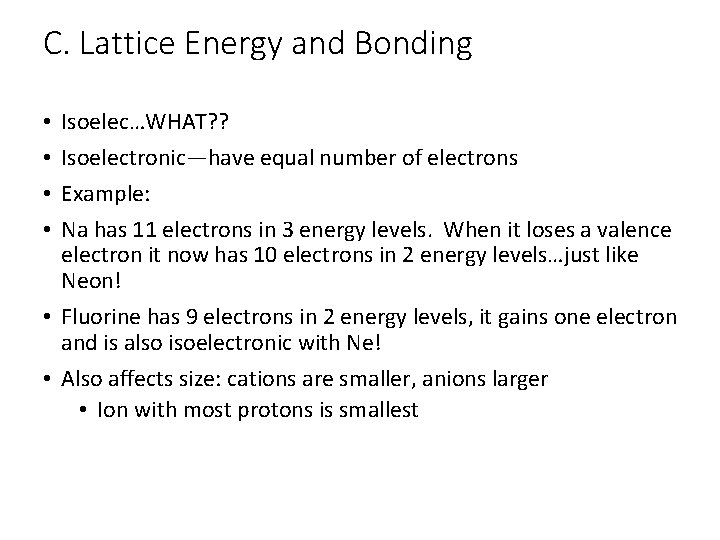C. Lattice Energy and Bonding Isoelec…WHAT? ? Isoelectronic—have equal number of electrons Example: Na has 11 electrons in 3 energy levels. When it loses a valence electron it now has 10 electrons in 2 energy levels…just like Neon! • Fluorine has 9 electrons in 2 energy levels, it gains one electron and is also isoelectronic with Ne! • Also affects size: cations are smaller, anions larger • Ion with most protons is smallest • •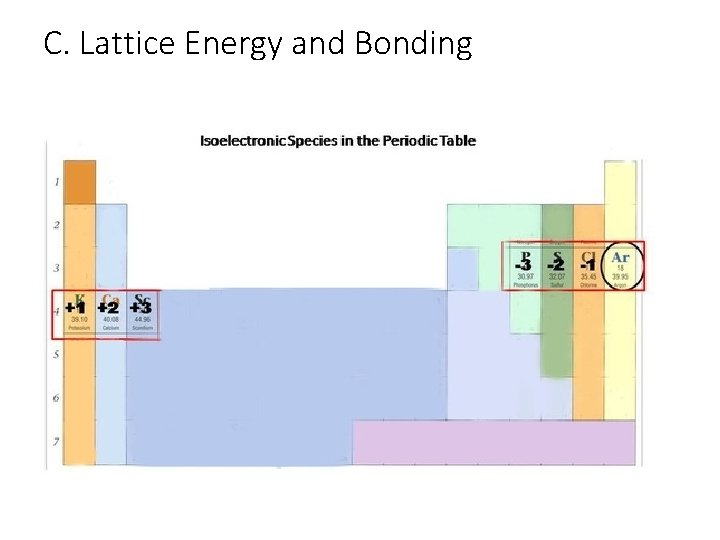C. Lattice Energy and BondingC. Lattice Energy and Bonding • Which has a stronger bond: Na. Cl or KCl? • Na. Cl • Na has stronger lattice energy so it has a stronger bond • Na+ has electrons in 2 energy levels, which is less than K+ (isoelectronic with Ar, e- in 3 energy levels)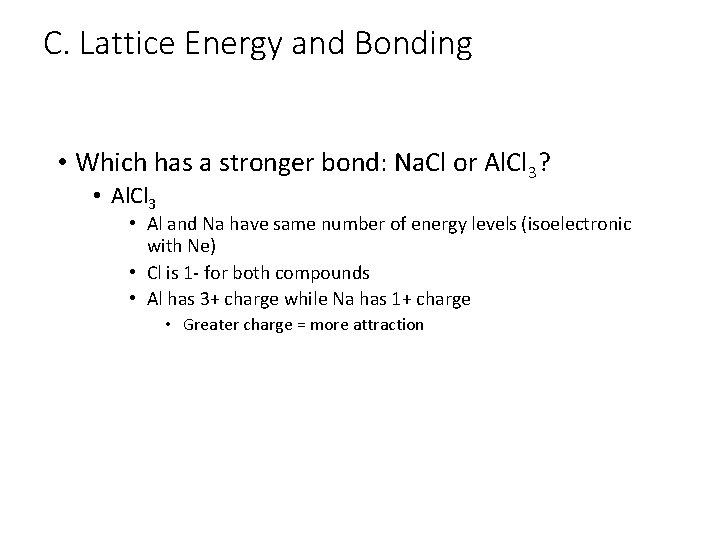C. Lattice Energy and Bonding • Which has a stronger bond: Na. Cl or Al. Cl 3? • Al. Cl 3 • Al and Na have same number of energy levels (isoelectronic with Ne) • Cl is 1 - for both compounds • Al has 3+ charge while Na has 1+ charge • Greater charge = more attraction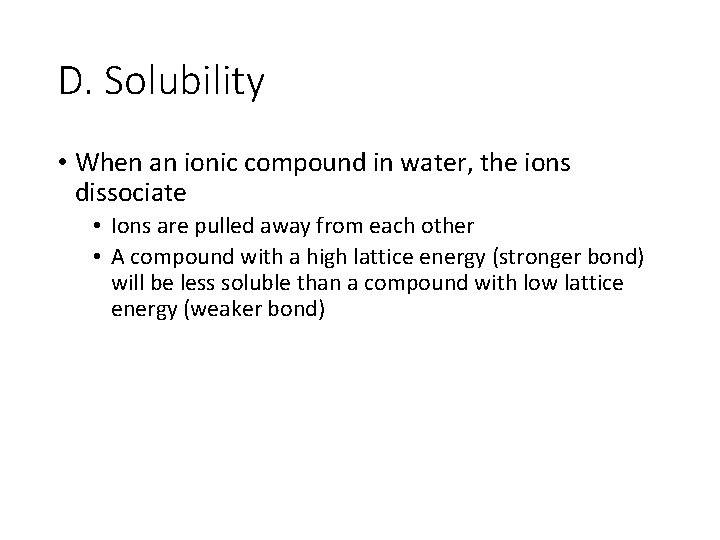D. Solubility • When an ionic compound in water, the ions dissociate • Ions are pulled away from each other • A compound with a high lattice energy (stronger bond) will be less soluble than a compound with low lattice energy (weaker bond)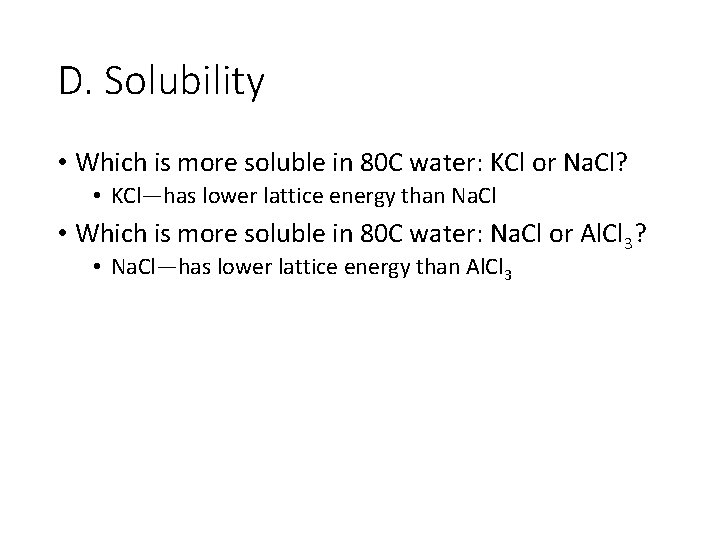D. Solubility • Which is more soluble in 80 C water: KCl or Na. Cl? • KCl—has lower lattice energy than Na. Cl • Which is more soluble in 80 C water: Na. Cl or Al. Cl 3? • Na. Cl—has lower lattice energy than Al. Cl 3